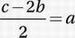# SAT Math Multiple Choice Question 726: Answer and Explanation

### Test Information

Question: 726

6. If the sum of a, b, and c is three times the sum of a and b, which of the following expresses the value of a in terms of b and c?

• A.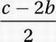• B.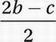• C.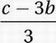• D.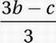Explanation:

A

Algebra (word problems) EASY

The sum of a, b, and c is three times the sum of a and b:

a + b + c = 3(a + b)

Distribute:

a + b + c = 3a + 3b

Subtract a:

b + c = 2a + 3b

Subtract 3b:

-2b + c = 2a

Divide by 2: